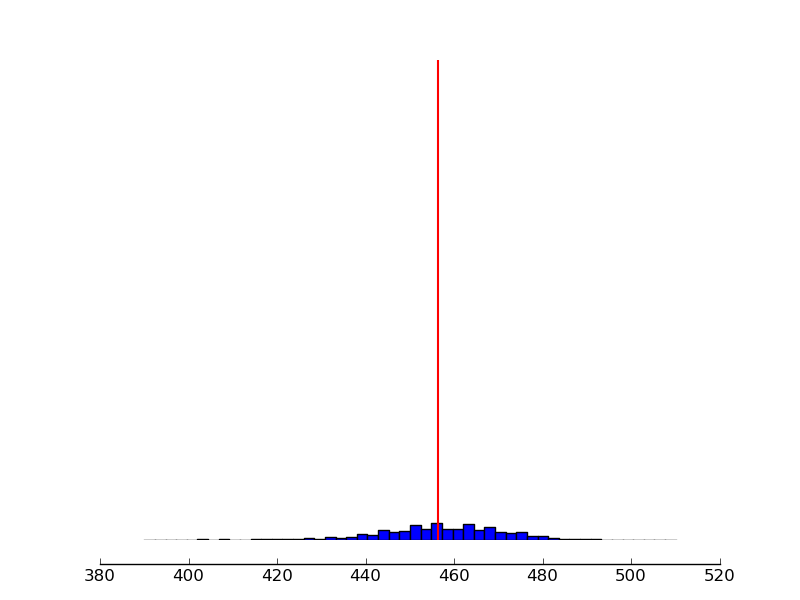# histogram and a line

Thank you for your solutions. However, with my dataset it did not work out with the following code:

import numpy as np

import matplotlib.pyplot as plt

with open(‘in.small.txt’) as f:

``````data = list(int(no.rstrip()) for no in f)

mean = np.mean(data)

print 'mean', mean

std = np.std(data)

print 'std', std

#fig = plt.figure(figsize=(8,6), dpi=72, facecolor='w')

fig = plt.figure()

axes = plt.subplot(111)

#axes.axvline(np.mean(data), 0, max(data), linewidth=2, color='red')

axes.axvline(x=mean, linewidth=1.5, color='r')

axes.hist(data, bins=50, normed=True)

#axes.set_ylim(0, 1)

#axes.set_xlim(-3, 3)

axes.spines['right'].set_color('none')

axes.spines['top'].set_color('none')

axes.xaxis.set_ticks_position('bottom')

axes.spines['bottom'].set_position(('data', -0.05))

axes.yaxis.set_ticks_position('left')

axes.spines['left'].set_position(('data',-3.25))

fig.savefig('myhist.png')
``````

Please find attached the histogram. The dataset I am sending with next email.···

On Fri, Mar 9, 2012 at 9:59 AM, Paul Hobson <pmhobson@…287…> wrote:

Check out the axvline method (of pyplot or an axes object). You’ll

only have to specify the x-value, and it’ll won’t rescale your y-axis.

See my edits below:

On Wed, Mar 7, 2012 at 12:28 AM, Mic <mictadlo@…287…> wrote:

Hello,

I am able to draw a histogram with the following code:

import matplotlib.pyplot as plt

hist_data = []

median = 249

f = plt.figure()

h.hist(hist_data, bins=50, normed=True)

h.axvline(x=median, linewidth=1.5, color=‘b’)

f.savefig(‘myhist.png’)

However, I don’t know how to draw a line for median at 249 position like in

attachment.

Virtualization & Cloud Management Using Capacity Planning

Cloud computing makes use of virtualization - but cloud computing

also focuses on allowing computing to be delivered as a service.

http://www.accelacomm.com/jaw/sfnl/114/51521223/

Matplotlib-users mailing list

Matplotlib-users@lists.sourceforge.net

https://lists.sourceforge.net/lists/listinfo/matplotlib-users

Mic :

``````    Thank you for your solutions. However,
``````

with my dataset it did not work out with the following code:

``````              Please find attached the histogram. The dataset I
``````

am sending with next email.

1. You haven’sent in.small.txt, but in.txt , 70 MB,
several millions lines. Please, be gentle wrt people who want to

1. You file is buggy, last two lines generate an exception. They
contain a text “mean …”.

2. I corrected them, the program works, and gives the picture you
attached. You don’t say WHAT is “not working”. Is it the question of
scaling, or something else?

4 If it is scaling, and IF IF … you want to obtain something I
attach, then use the code:

`hst=axes.hist(data, bins=50, normed=True) mxh=max(hst) plt.plot([mean,mean],[0,mxh],'r',lw=2)`
5. Learn something about WHAT is a normed histogram in matplotlib,
how to get into the plotted data, and to adjust the scales.
Otherwise such problems will repeat.

``````6. Finally, in our civilized world people sign their messages.
``````

Anonymous letters are ugly.

``Jerzy Karczmarczuk``